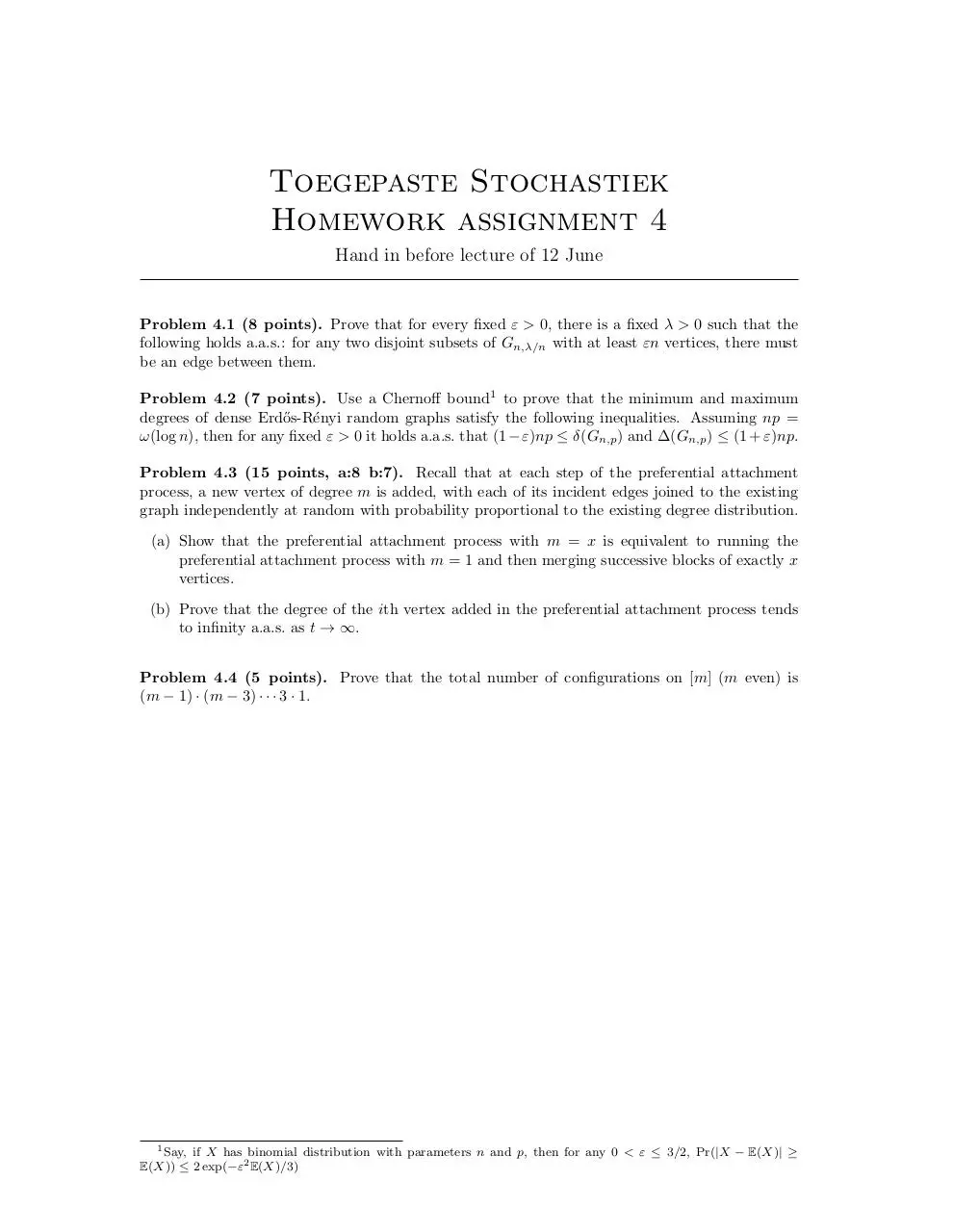# HW4 .pdf

### File information

This PDF 1.5 document has been generated by TeX / pdfTeX-1.40.14, and has been sent on pdf-archive.com on 03/06/2014 at 20:40, from IP address 89.98.x.x. The current document download page has been viewed 525 times.
File size: 127.39 KB (1 page).
Privacy: public file

### Document preview

Toegepaste Stochastiek
Homework assignment 4
Hand in before lecture of 12 June

Problem 4.1 (8 points). Prove that for every fixed ε &gt; 0, there is a fixed λ &gt; 0 such that the
following holds a.a.s.: for any two disjoint subsets of Gn,λ/n with at least εn vertices, there must
be an edge between them.
Problem 4.2 (7 points). Use a Chernoff bound1 to prove that the minimum and maximum
degrees of dense Erd˝
os-R´enyi random graphs satisfy the following inequalities. Assuming np =
ω(log n), then for any fixed ε &gt; 0 it holds a.a.s. that (1 − ε)np ≤ δ(Gn,p ) and ∆(Gn,p ) ≤ (1 + ε)np.
Problem 4.3 (15 points, a:8 b:7). Recall that at each step of the preferential attachment
process, a new vertex of degree m is added, with each of its incident edges joined to the existing
graph independently at random with probability proportional to the existing degree distribution.
(a) Show that the preferential attachment process with m = x is equivalent to running the
preferential attachment process with m = 1 and then merging successive blocks of exactly x
vertices.
(b) Prove that the degree of the ith vertex added in the preferential attachment process tends
to infinity a.a.s. as t → ∞.
Problem 4.4 (5 points). Prove that the total number of configurations on [m] (m even) is
(m − 1) · (m − 3) · · · 3 · 1.

1 Say, if X has binomial distribution with parameters n and p, then for any 0 &lt; ε ≤ 3/2, Pr(|X − E(X)| ≥
E(X)) ≤ 2 exp(−ε2 E(X)/3)HW4.pdf (PDF, 127.39 KB)

### Share on social networks

#### HTML Code

Copy the following HTML code to share your document on a Website or Blog